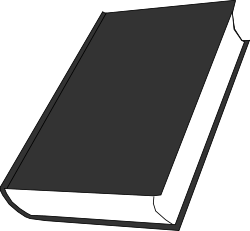Problems
113 - Profit Percentage

Profit Percentage

Time Limit: 1 sec

The Problem

A book is bought for X taka and sold for Y taka. What is the percentage profit ?

[Note: here Y > X]

Example:

if X = 50, and Y = 55 then

The percentage profit is 10 taka.The Input

The input file contains only 2 integer numbers X, Y (X < 10000<= Y <= 30000).

The Output

Output will show the one integer numbers which is profit percentage in a separate line.

Sample Input

50 55

Sample Output

10

Problem Setter: Shahin ShamS